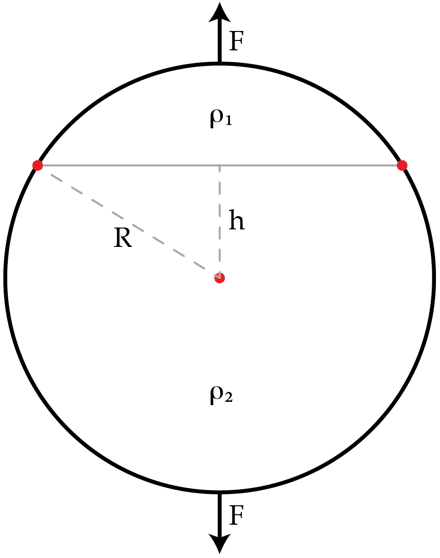# Combining charged caps

Two spherical caps with constant volume charge densities $\rho_{1}$ and $\rho_{2}$ are in contact and form a ball or radius R as shown in the figure. Find the force of interaction $F$ between the caps in Newtons if $\rho_{1}=1\times 10^{-3}~\text{C}/\text{m}^{3}$, $\rho_{2}=2\times 10^{-3}~\text{C}/\text{m}^{3}$, $R=10~\text{cm}$ and $h=5~\text{cm}$.Details and assumptions

The vacuum permittivity is $\varepsilon_{0}=8.85\times 10^{-12}~\text{F}/\text{m}$.

×

Problem Loading...

Note Loading...

Set Loading...